# Graph the equation with the help of graphing calculator### Precalculus: Mathematics for Calcu...

6th Edition
Stewart + 5 others
Publisher: Cengage Learning
ISBN: 9780840068071### Precalculus: Mathematics for Calcu...

6th Edition
Stewart + 5 others
Publisher: Cengage Learning
ISBN: 9780840068071

#### Solutions

Chapter 1.9, Problem 74E
To determine

## Graph the equation with the help of graphing calculator

Expert Solution

### Explanation of Solution

Given information:

Write a short essay describing different ways in which a graphing calculator might give a misleading graph of an equation.

Calculation:

A graphing calculator displays a rectangular portion of the graph of an equation in a display window or viewing screen, which we call a viewing rectangle. The default screen often gives an incomplete or misleading picture, so it is important to coose the viewing rectangle with care.

Let us graph the equation y=x2+3 with different viewing rectangles. We start with the viewing rectangle [2,2]by[2,2] . The resulting graph as shown below is blank.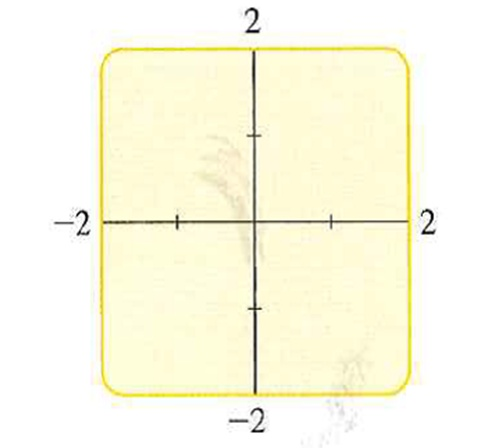This is because for all x ,

x20x2+33

Hence, the graph lies entirely above the viewing rectangle, so this viewing rectangle is not appropriate.

If we enlarge the viewing rectangle to [4,4]by[4,4] , as illustrated below, we begin to see a portion of the graph.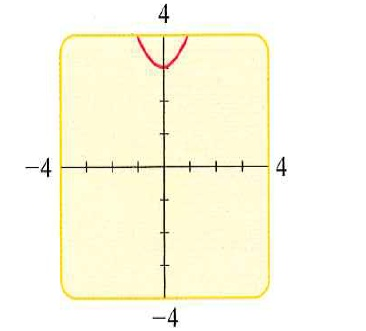If we enlarge the viewing rectangle to [10,10]by[5,30] , the graph seems to be more complete.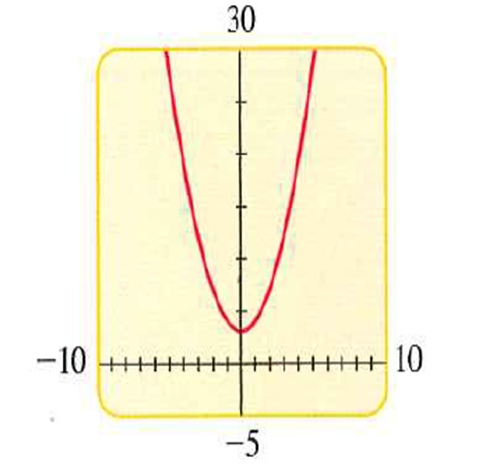Further enlarging the viewing rectangle, the graph do not show the y intercept is 3 .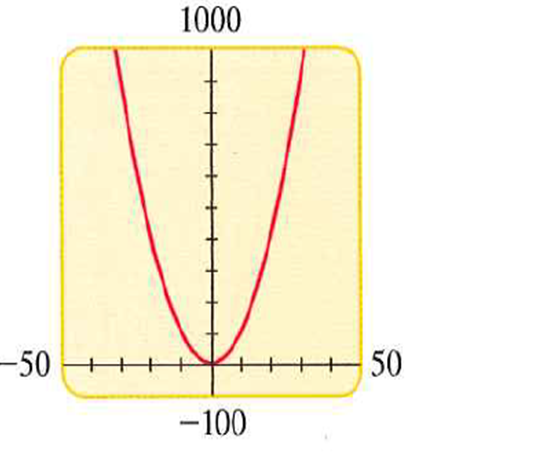Hence, the viewing rectangle to [10,10]by[5,30] is an appropriate representation of the graph.

Now graph the equations y=3x26+1&y=0.23x2.25 together in the viewing rectangle [1,3]by[2.5,1.5] . The graphs are so shown below.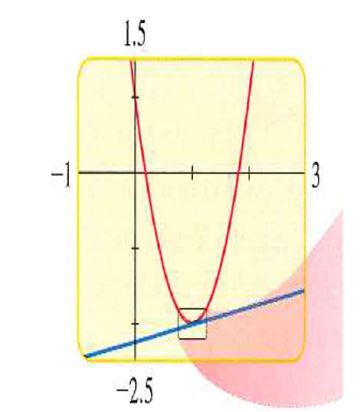One is a parabola, while the other is a line. It seems as if the graphs intersect near the point (1,2) .

Hence, if we zoom in on the area around this point as illustrated below, we see that although the graphs almost touch, but they do not actually intersect.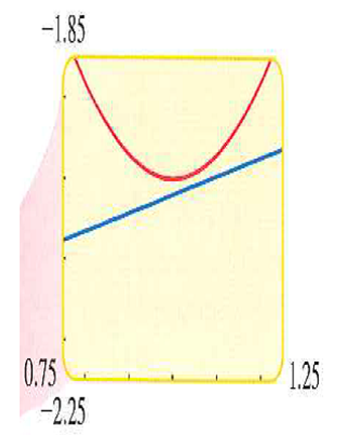### Have a homework question?

Subscribe to bartleby learn! Ask subject matter experts 30 homework questions each month. Plus, you’ll have access to millions of step-by-step textbook answers!